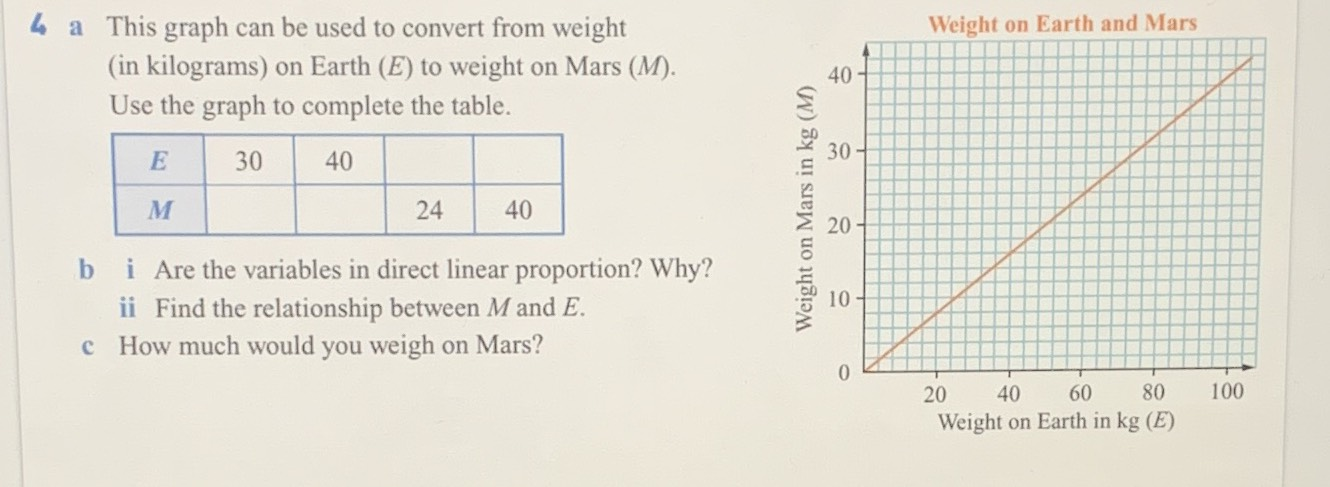### ¿Todavía tienes preguntas de matemáticas?

Pregunte a nuestros tutores expertos
Algebra
Pregunta$$4$$ a This graph can be used to convert from weight (in kilograms) on Earth $$( E )$$ to weight on Mars $$( M )$$ . Use the graph to complete the table.

b i Are the variables in direct linear proportion? Why?

ii Find the relationship between $$M$$ and $$E$$ .

c How much would you weigh on Mars?

$$60$$      $$100$$
$$12$$       $$16$$